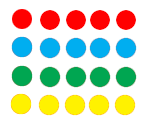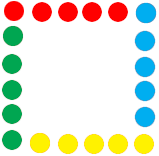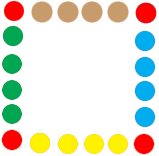SEARCH HOMEMath Central Quandaries & QueriesQuestion from Angela: Following is a problem from my 3rd grader's homework assignment: Solve. Draw a diagram to represent the situation. Rachel has 20 one-inch beads. She wants to use all of them to make a square picture frame. What will be the length of each side? Find Perimeter, Area, and Side LengthAngela continued:

At first glance, this problem looks as though it’s a simple matter of division. A square has 4 equal sides so: 20 ÷ 4 = 5. Therefore, each side has 5 beads.
However the problem also states that Rachel wants to use all the beads to make a square picture frame.
Here’s the problem with each side having 5 beads:
BBBBB
B      B
B      B
B      B
BBBBB
Counting the total number of beads used at 16, Rachel has not used all 20. To use all 20 each side would have to have 6 beads instead.
BBBBBB
B       B
B       B
B       B
B       B
BBBBBB

How would this problem be solved mathematically on a 3rd grade level?

Hi Angela,

Many times in solving a problem you try something, it doesn't work but it shows you how to correct your solution. In your first attempt the four corner beads got counted twice. For example the bead in the upper left corner is counted because it is in the top of the frame and also because it is the left side of the frame.

BBBBB
B      B
B      B
B      B
BBBBB

One way to correct this is to divide 20 by 4 as you did and then take the 4 collections of 5 beadsand arrange them to form the sides of the picture frameIf you realize from your first attempt that the corners are the problem you could start by placing the four corners and then take the 16 remaining beads, divide by 4 to get 4 and then fill in the four sides with 4 beads each.I hope this helps,
PennyMath Central is supported by the University of Regina and The Pacific Institute for the Mathematical Sciences.